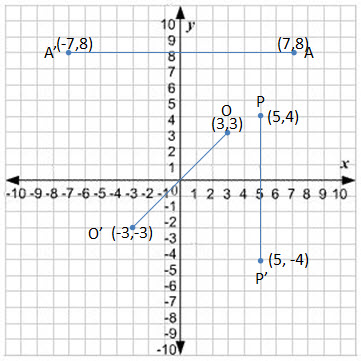You could also refer to some notes on coordinate geometry published before.

Things to remember:

1. In A Co-ordinate plane, there are two axis:$x-axis$ and$y-axis$.
2. Any point on a plane can be represented as$(x, y)$
3. When you state the coordinate of a point, the abscissa ($x$ coordinate) precedes the ordinate ($y$ coordinate).
4. Co-ordinate of origin is$(0, 0)$
5. Co-ordinate of a point of$x-axis \ is \ (x,0)$
6. Co-ordinate of a point of$y-axis \ is \ (y,0)$

Reflection:When any object is placed in front of a mirror, its image is formed at the same distance behind the mirror as the object is front of it.

Here$OP = OP'$ also$\angle BOP = \angle AOP' = 90^{\circ}$

Reflection in line$y-axis$
In the adjoining diagram, you see that point$A's$ reflection is$A'$. The$y-coordinate$ does not change, only the$x-coordinate$ changes.$(7, 8)$ become$(-7, 8)$ when reflected on$y-axis$.

In a generic form, we can say that the reflection of$(x,y)$on$y-axis$ is$(-x,y)$.

Reflection in line$x-axis$

Similarly, you see that point$P's$ reflection is$P'$. The$x-coordinate$ does not change, only the$y-coordinate$ changes.$(5, 4)$ become$(5, -4)$ when reflected on$y-axis$.

In a generic form, we can say that the reflection of$(x,y)$ on$x-axis$ is$(x,-y)$

Reflection in the origin:

In the above diagram, you would see that in reflection in Origin, both$x-coordinate$ and$y-coordinate$ change.$(3, 3)$ become$(-3, -3)$ when reflected in the origin.

Invariant Point:

Any point that remains unaltered under a given transformation is called invariant. For example:

1. Reflection of$(5,0)$ in$x-axis$ would remain same as$(5,0)$
2. Reflection of$(0,5)$ in$y-axis$ would remain same as$(0,5)$
3. Reflection of$(0,0)$ in$origin$ would remain same as$(0,0)$

Remember, in case of an Invariant point, the point itself is its own image. Similarly, every point$P$ in a like$AB$ is reflected in$AB$ itself , the point is invariant.